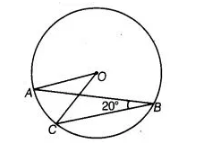# In figure, if ∠ABC = 20°,`
Question:

In figure, if $\angle A B C=20^{\circ}$, then $\angle A O C$ is equal to(a) $20^{\circ}$

(b) $40^{\circ}$

(c) $60^{\circ}$

(d) $10^{\circ}$

Thinking Process

Use the theorem, that in a circale the angle subtended by an arc at the centre is twice the angle subtended by it at the remaining part of the circle and

further simplify it.

Solution:

(b) Given, ∠ABC = 20°

We know that, angle subtended at the centre by an arc is twice the angle subtended by it at the remaining part of circle.

∠AOC = 2∠ABC = 2 x 20° = 40°